# Introducing General Recursion CS 5010 Program Design Paradigms

• Slides: 35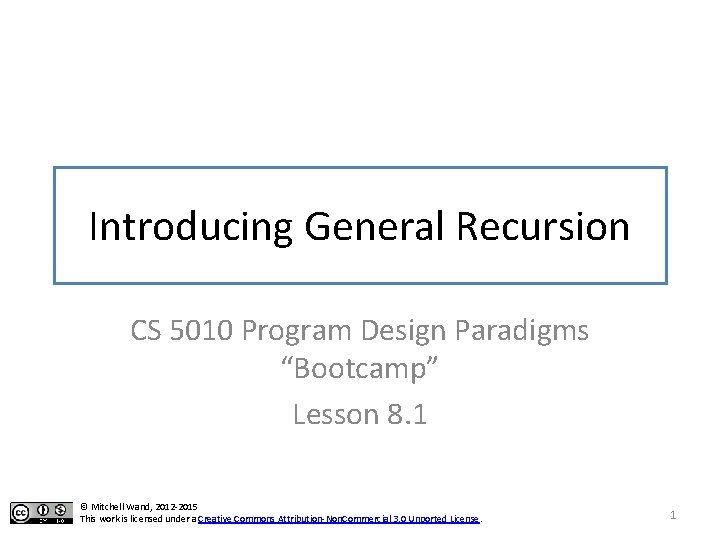Module Introduction • So far, we've written our functions using the destructor template to recur on the sub-pieces of the data. We sometimes call this structural recursion. • In this module, we'll see some examples of problems that don't fit neatly into this pattern. • We'll introduce a new family of strategies, called general recursion, to describe these examples. • General recursion and invariants together provide a powerful combination. 2Module 08 Data Representations Design Strategies Basics Combine simpler functions Generalization Mixed Data Use a template Over Constants Recursive Data Divide into Cases Over Expressions Functional Data Call a more general function Over Contexts Objects & Classes Recur on subproblem Over Data Representations Stateful Objects Communicate via State Over Method Implementations 3Structural Recursion • Our destructor templates always recurred on the sub-pieces of our structure. • We call this structural recursion. • Let's look at an example that doesn't fit into this mold. 4An example: decode (define-struct diffexp (exp 1 exp 2)) ; ; A Diff. Exp is either ; ; -- a Number ; ; -- (make-diffexp Diff. Exp) Here is the data definition for diffexps. These are a simple representation of difference expressions, much like the arithmetic expressions we considered in some of the earlier problem sets. 5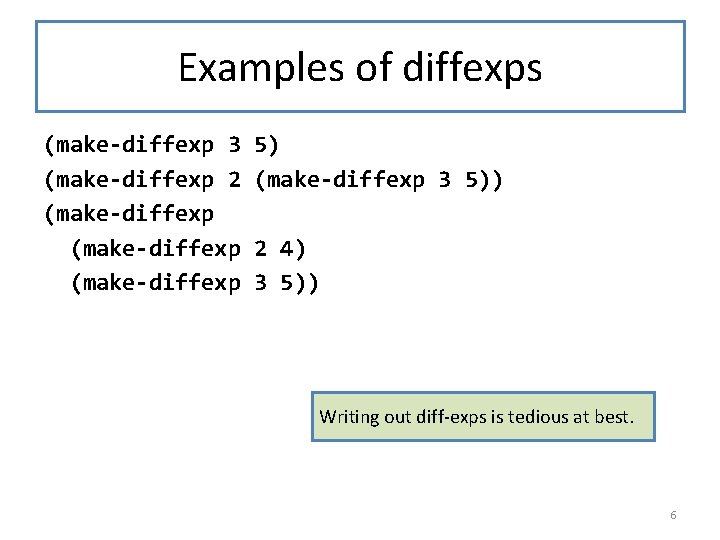Examples of diffexps (make-diffexp 3 (make-diffexp 2 (make-diffexp 5) (make-diffexp 3 5)) 2 4) 3 5)) Writing out diff-exps is tedious at best. 6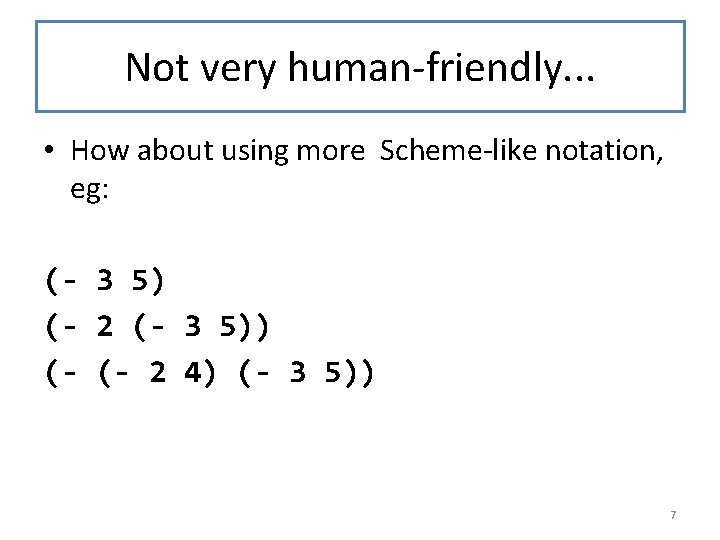Not very human-friendly. . . • How about using more Scheme-like notation, eg: (- 3 5) (- 2 (- 3 5)) (- (- 2 4) (- 3 5)) 7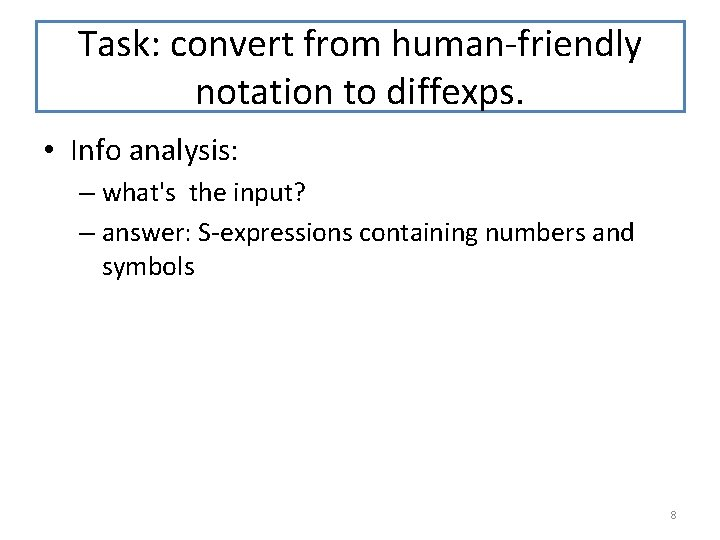Task: convert from human-friendly notation to diffexps. • Info analysis: – what's the input? – answer: S-expressions containing numbers and symbols 8Data Definitions ; ; An Atom is one of ; ; -- a Number ; ; -- a Symbol Here is a formal data definition for the inputs to our function. ; ; An Sexp. Of. Atom is either ; ; -- an Atom ; ; -- a List. Of. Sexp. Of. Atom ; ; A List. Of. Sexp. Of. Atom is either ; ; -- empty ; ; -- (cons Sexp. Of. Atom List. Of. Sexp. Of. Atom) 9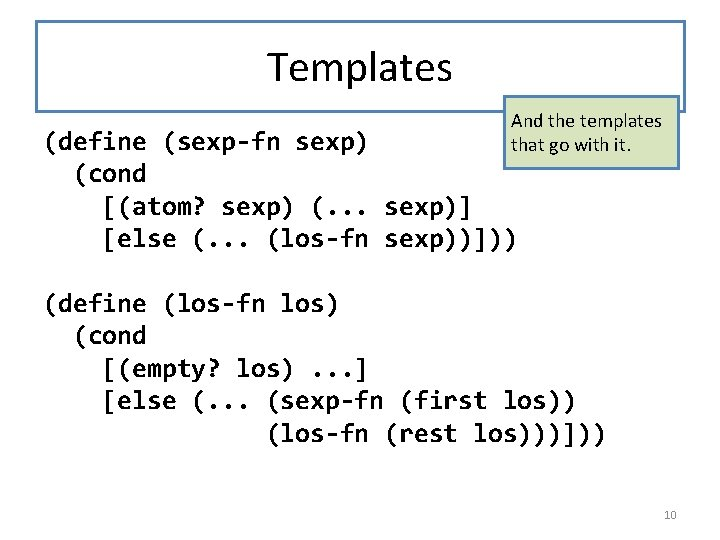Templates And the templates that go with it. (define (sexp-fn sexp) (cond [(atom? sexp) (. . . sexp)] [else (. . . (los-fn sexp))])) (define (los-fn los) (cond [(empty? los). . . ] [else (. . . (sexp-fn (first los)) (los-fn (rest los)))])) 10Contract and Examples decode : Sexp. Of. Atom -> Diff. Exp (- 3 5) => (make-diffexp 3 5) (- 2 (- 3 5)) => (make-diffexp 2 (make-diffexp 3 5)) (- (- 2 4) (- 3 5)) => (make-diffexp 2 4) (make-diffexp 3 5)) 11Umm, but not every Sexp. Of. Atom corresponds to a diffexp (- 3) (+ 3 5) (- (+ 3 5) 5) ((1)) ((- 2 3) (- 1 0)) (- 3 5 7) does does not not not correspond correspond to to to any any any diffexp diffexp But here are some other inputs that are legal inputs according to our contract. None of these is the human-friendly representation of any diff-exp. 12A Better Contract ; ; A Maybe. X is one of ; ; -- false ; ; -- X ; ; (define (maybex-fn mx) ; ; (cond ; ; [(false? mx). . . ] ; ; [else (. . . mx)])) To account for this, we change our contract to produce a Maybe. Diff. Exp instead of a Diff. Exp. If the Sexp. Of. Atom doesn't correspond to any Diff. Exp, we'll have our decode function return false. decode : Sexp. Of. Atom -> Maybe. Diff. Exp 13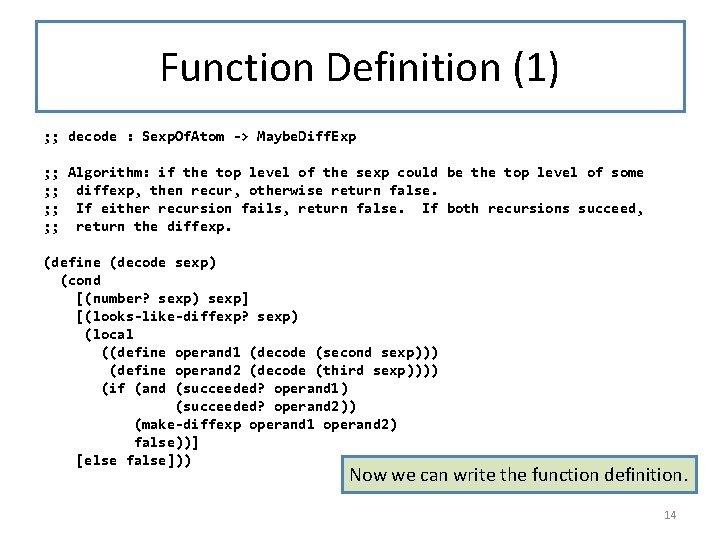Function Definition (1) ; ; decode : Sexp. Of. Atom -> Maybe. Diff. Exp ; ; Algorithm: if the top level of the sexp could be the top level of some ; ; diffexp, then recur, otherwise return false. ; ; If either recursion fails, return false. If both recursions succeed, ; ; return the diffexp. (define (decode sexp) (cond [(number? sexp) sexp] [(looks-like-diffexp? sexp) (local ((define operand 1 (decode (second sexp))) (define operand 2 (decode (third sexp)))) (if (and (succeeded? operand 1) (succeeded? operand 2)) (make-diffexp operand 1 operand 2) false))] [else false])) Now we can write the function definition. 14Function Definition (2) ; ; looks-like-diffexp? : Sexp. Of. Atom -> Boolean ; ; WHERE: sexp is not a number. ; ; RETURNS: true iff the top level of the sexp could be the top ; ; level of some diffexp. ; ; At the top level, a representation of a ; ; diffexp must be either a number or a list of ; ; exactly 3 elements, beginning with the symbol ; ; STRATEGY: combine simpler functions (define (looks-like-diffexp? sexp) (and In this function definition, we add an invariant (the WHERE clause) (list? sexp) to record the assumption that our ; ; at this point we know that input is not merely an ; ; sexp is a list Sexp. Of. Atom, but is rather an (= (length sexp) 3) Sexp. Of. Atom that is not a number. (equal? (first sexp) '-))) We know this assumption is true, because looks-like-diffexp? is only called after number? fails. 15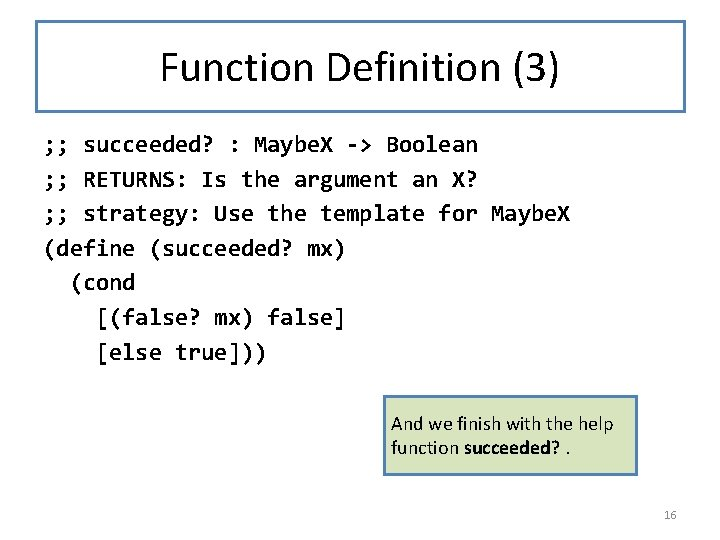Function Definition (3) ; ; succeeded? : Maybe. X -> Boolean ; ; RETURNS: Is the argument an X? ; ; strategy: Use the template for Maybe. X (define (succeeded? mx) (cond [(false? mx) false] [else true])) And we finish with the help function succeeded? . 16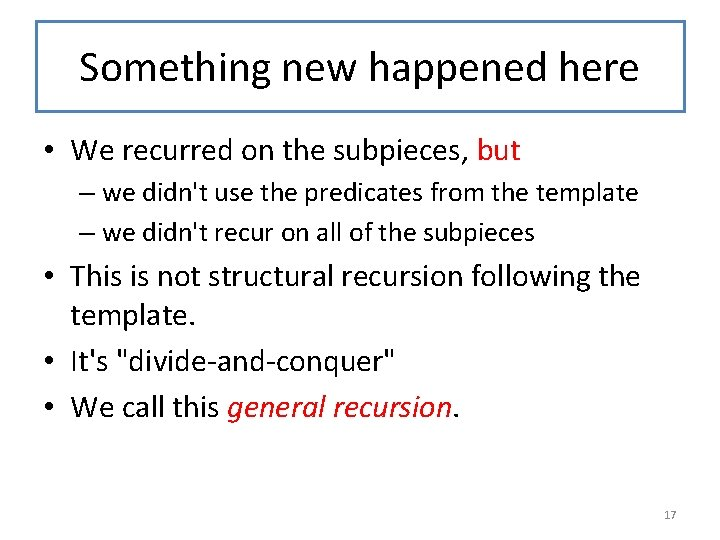Something new happened here • We recurred on the subpieces, but – we didn't use the predicates from the template – we didn't recur on all of the subpieces • This is not structural recursion following the template. • It's "divide-and-conquer" • We call this general recursion. 17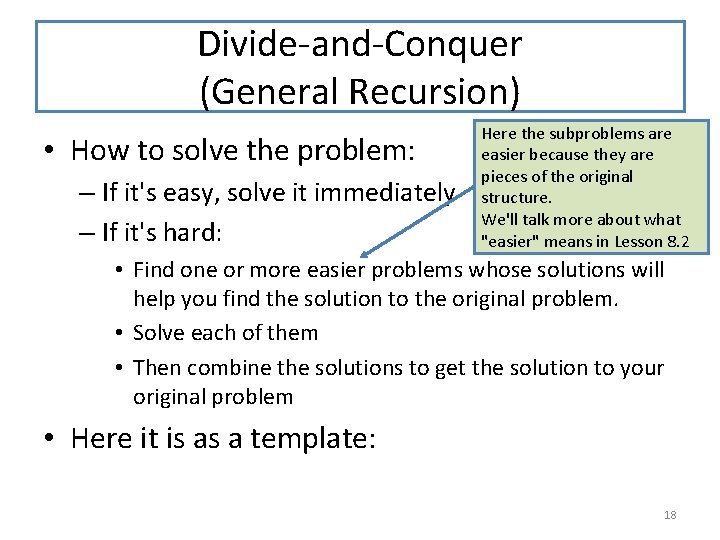Divide-and-Conquer (General Recursion) • How to solve the problem: – If it's easy, solve it immediately – If it's hard: Here the subproblems are easier because they are pieces of the original structure. We'll talk more about what "easier" means in Lesson 8. 2 • Find one or more easier problems whose solutions will help you find the solution to the original problem. • Solve each of them • Then combine the solutions to get the solution to your original problem • Here it is as a template: 18Pattern for General Recursion (1) ; ; solve : Problem -> Solution Instead of using ellipses (". . . "'s), ; ; purpose statement. . . we've give each slot a name ; ; TERMINATION ARGUMENT: explain why new-problem 1 and new(displayed in orange) so you can problem 2 are easier than the-problem. see the role it plays. (define (solution the-problem) (cond [(trivial 1? the-problem) (trivial-solution 1 the-problem)] [(trivial 2? the-problem) (trivial-solution 2 the-problem)] [(difficult? the-problem) (local ((define solution 1 (solve (simpler-instance 1 the-problem))) (define solution 2 (solve (simpler-instance 2 the-problem)))) (combine-solutions solution 1 solution 2))])) There is no magic recipe for finding easier subproblems. You must understand the 19 structure of the problem domain.There's more than one pattern • The pattern might take different shapes, depending on our problem. • We might have different numbers of trivial cases, or different numbers of subproblems. • Let's write this down as a recipe, and then look at some of the possibilities. 20The General Recursion Recipe Question Answer 1. Are there different cases of your problem, each with a different kind of solution? Write a cond with a clause for each case. 2. How do the cases differ from each other? Use the differences to formulate a condition per case 3. For each case: a. b. c. d. Identify one or more instances of your problem that are simpler than the original. Document why they are simpler Extract each instance and recur to solve it. Combine the solutions of your easier instances to get a solution to your original problem. 21Writing down your strategy We’ll write down our strategies as things like STRATEGY: Recur on <value> or STRATEGY: Recur on <value>; halt when <condition> or STRATEGY: Recur on <values>; <describe how answers are combined> That’s pretty vague– we’ll see more as we do more examples. 22Another General-Recursion Pattern ; ; solve : Problem -> Solution ; ; STRATEGY: Recur on simpler-instance ARGUMENT: explain why new -problem 1 and new-problem 2 are easier than the-problem. (define (solution the-problem) (cond [(trivial 1? the-problem) (trivial-solution 1 the-problem)] [(trivial 2? the-problem) (trivial-solution 2 the-problem)] [(difficult? the-problem) (local ((define solution 1 (solve (simpler-instance the-problem)))) (adapt-solution 1))])) Here's a version with two trivial cases and one difficult case, where simpler-instance : Problem -> Problem the difficult case involves only one adapt-solution : Solution -> Solution subproblem. Most of our functions involving lists match this pattern. 23Yet Another General-Recursion Pattern ; ; solve : Problem -> Solution ; ; STRATEGY: Recur on (generate-subproblems the-problem), then use adapt-solutions TERMINATION ARGUMENT: explain why new-problem (define (solution the-problem) (cond [(trivial 1? the-problem) (trivial-solution 1 the-problem)] [(trivial 2? the-problem) (trivial-solution 2 the-problem)] [(difficult? the-problem) (local ((define new-problems (generate-subproblems the-problem))) (adapt-solutions Here's a version where (map solve new-problems))])) the difficult case generate-subproblem : Problem -> List. Of. Problem adapt-solutions : List. Of. Solution -> Solution requires solving a whole list of subproblems. A tree where a node has a list of sons may lead to use of this pattern. 24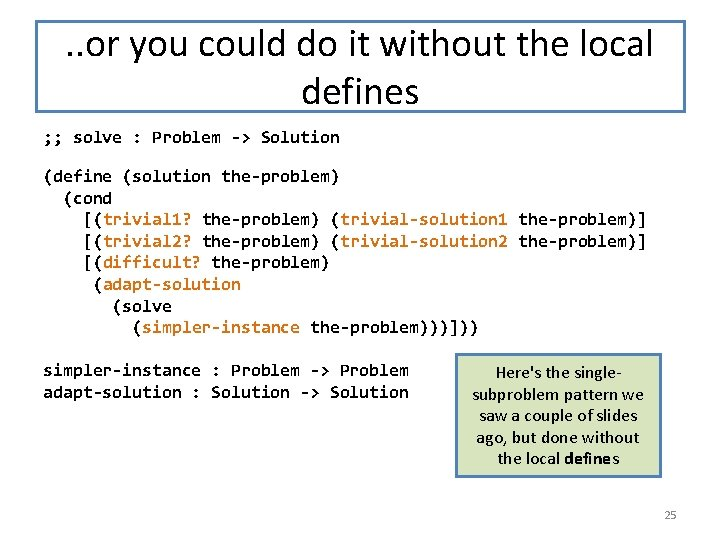. . or you could do it without the local defines ; ; solve : Problem -> Solution ARGUMENT: explain why newproblem 1 and new-problem 2 are easier than the-problem. (define (solution the-problem) (cond [(trivial 1? the-problem) (trivial-solution 1 the-problem)] [(trivial 2? the-problem) (trivial-solution 2 the-problem)] [(difficult? the-problem) (adapt-solution (solve (simpler-instance the-problem)))])) simpler-instance : Problem -> Problem adapt-solution : Solution -> Solution Here's the singlesubproblem pattern we saw a couple of slides ago, but done without the local defines 25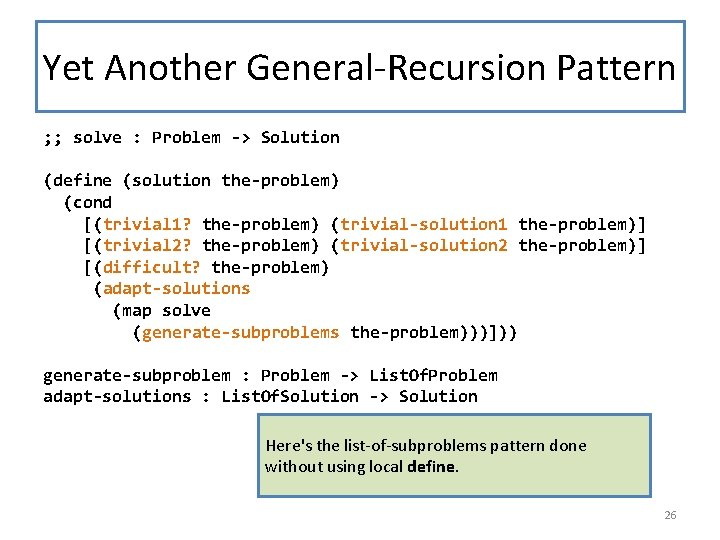Yet Another General-Recursion Pattern ; ; solve : Problem -> Solution TERMINATION ARGUMENT: explain why new-problem (define (solution the-problem) (cond [(trivial 1? the-problem) (trivial-solution 1 the-problem)] [(trivial 2? the-problem) (trivial-solution 2 the-problem)] [(difficult? the-problem) (adapt-solutions (map solve (generate-subproblems the-problem)))])) generate-subproblem : Problem -> List. Of. Problem adapt-solutions : List. Of. Solution -> Solution Here's the list-of-subproblems pattern done without using local define. 26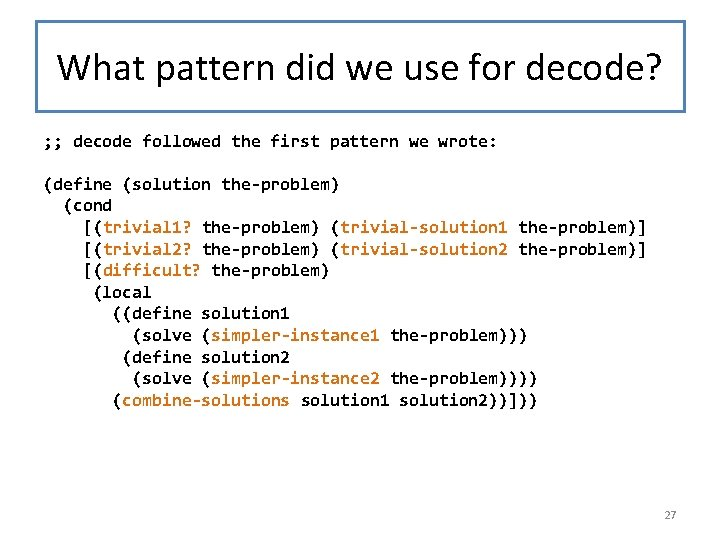What pattern did we use for decode? ; ; decode followed the first pattern we wrote: (define (solution the-problem) (cond [(trivial 1? the-problem) (trivial-solution 1 the-problem)] [(trivial 2? the-problem) (trivial-solution 2 the-problem)] [(difficult? the-problem) (local ((define solution 1 (solve (simpler-instance 1 the-problem))) (define solution 2 (solve (simpler-instance 2 the-problem)))) (combine-solutions solution 1 solution 2))])) 27Writing this down for decode ; ; decode : Sexp. Of. Atom -> Maybe. Diff. Exp ; ; STRATEGY: if the top level of sexp could be the top level of ; ; a diffexp, recur on 2 nd and 3 rd elements. (define (decode sexp) (cond [(number? sexp) sexp] [(could-be-diffexp? sexp) (local ((define operand 1 (decode (second sexp))) (define operand 2 (decode (third sexp)))) (if (and (succeeded? operand 1) (succeeded? operand 2)) (make-diffexp operand 1 operand 2) false))] The [else false])) strategy is a tweet-sized description of how the function works. We’ll see more about this later. 28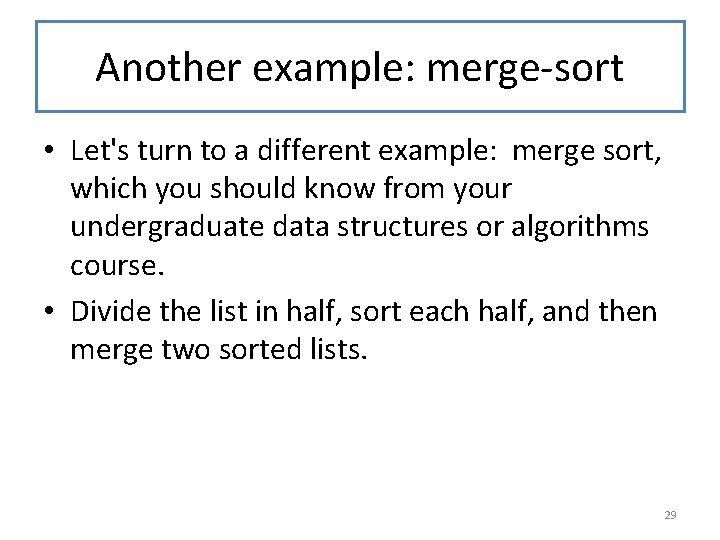Another example: merge-sort • Let's turn to a different example: merge sort, which you should know from your undergraduate data structures or algorithms course. • Divide the list in half, sort each half, and then merge two sorted lists. 29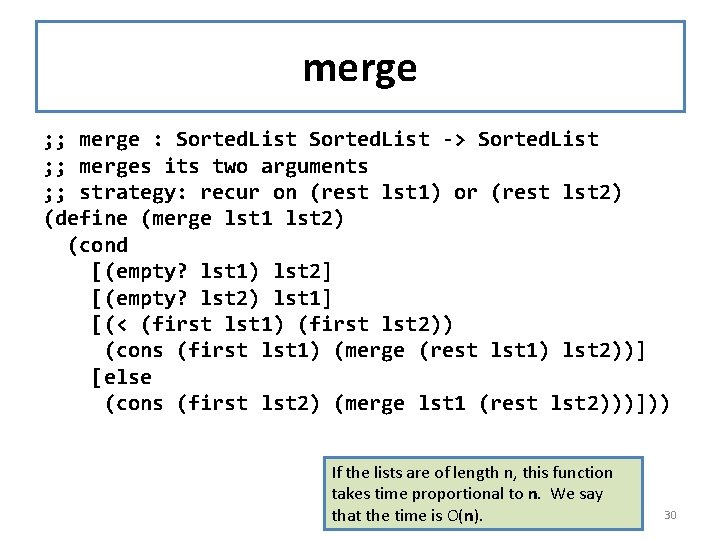merge ; ; merge : Sorted. List -> Sorted. List ; ; merges its two arguments ; ; strategy: recur on (rest lst 1) or (rest lst 2) (define (merge lst 1 lst 2) (cond [(empty? lst 1) lst 2] [(empty? lst 2) lst 1] [(< (first lst 1) (first lst 2)) (cons (first lst 1) (merge (rest lst 1) lst 2))] [else (cons (first lst 2) (merge lst 1 (rest lst 2)))])) If the lists are of length n, this function takes time proportional to n. We say that the time is O(n). 30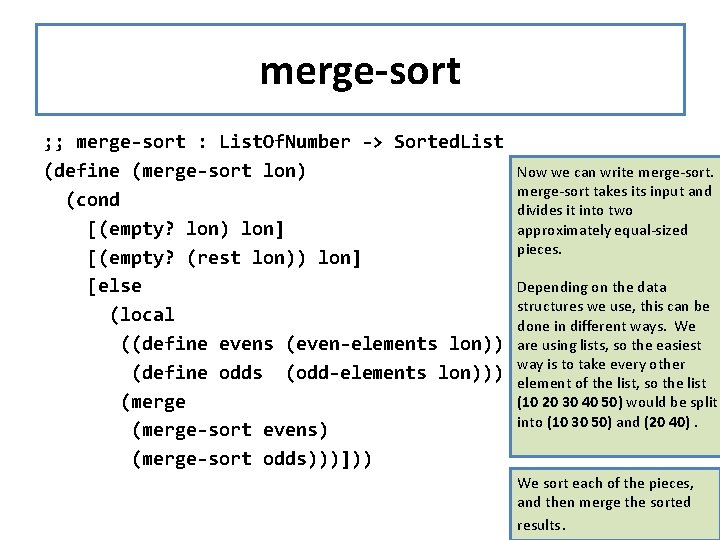merge-sort ; ; merge-sort : List. Of. Number -> Sorted. List (define (merge-sort lon) (cond [(empty? lon) lon] [(empty? (rest lon)) lon] [else (local ((define evens (even-elements lon)) (define odds (odd-elements lon))) (merge-sort evens) (merge-sort odds)))])) Now we can write merge-sort takes its input and divides it into two approximately equal-sized pieces. Depending on the data structures we use, this can be done in different ways. We are using lists, so the easiest way is to take every other element of the list, so the list (10 20 30 40 50) would be split into (10 30 50) and (20 40). We sort each of the pieces, and then merge the sorted 31 results.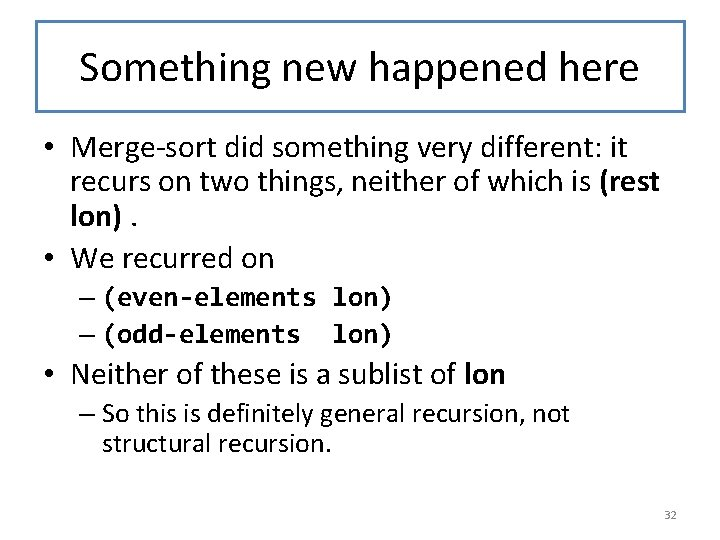Something new happened here • Merge-sort did something very different: it recurs on two things, neither of which is (rest lon). • We recurred on – (even-elements lon) – (odd-elements lon) • Neither of these is a sublist of lon – So this is definitely general recursion, not structural recursion. 32Running time for merge sort • Splitting the list in this way takes time proportional to the length n of the list. The call to merge likewise takes time proportional to n. We say this time is O(n). • If T(n) is the time to sort a list of length n, then T(n) is equal to the time 2*T(n/2) that it takes to sort the two sublists, plus the time O(n) of splitting the list and merging the two results: • So the overall time is T(n) = 2*T(n/2) + O(n) • When you take algorithms, you will learn that all this implies that T(n) = O(n log n). This is better than an insertion sort, which takes O(n^2). 33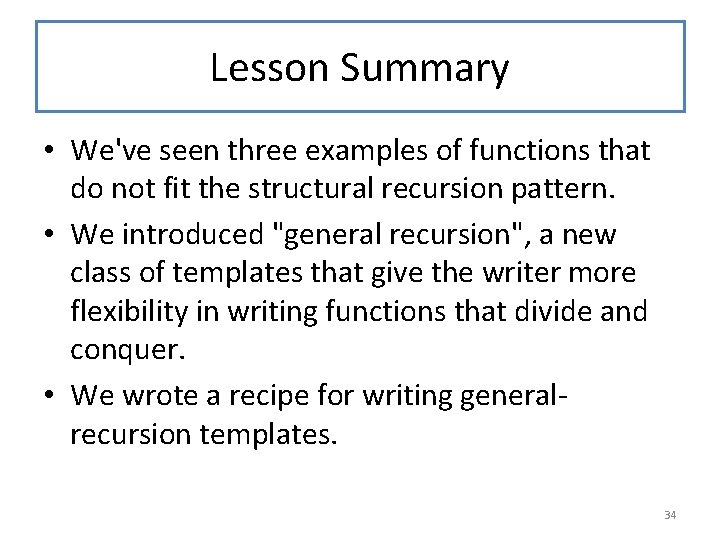Lesson Summary • We've seen three examples of functions that do not fit the structural recursion pattern. • We introduced "general recursion", a new class of templates that give the writer more flexibility in writing functions that divide and conquer. • We wrote a recipe for writing generalrecursion templates. 34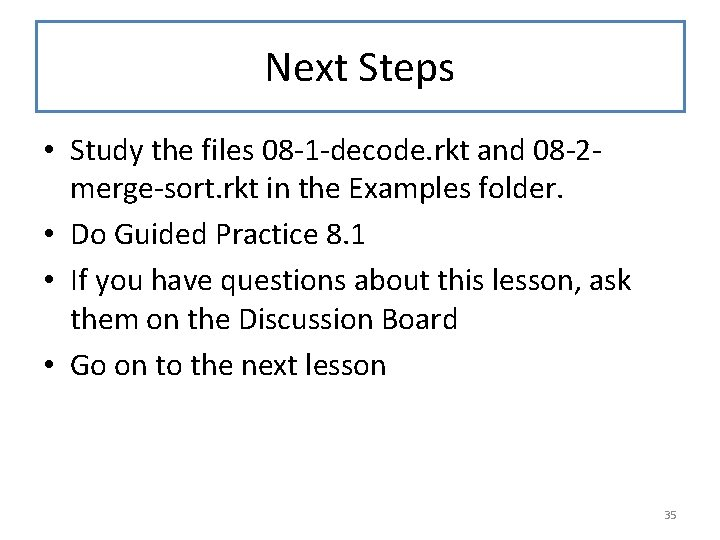Next Steps • Study the files 08 -1 -decode. rkt and 08 -2 merge-sort. rkt in the Examples folder. • Do Guided Practice 8. 1 • If you have questions about this lesson, ask them on the Discussion Board • Go on to the next lesson 35